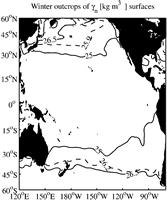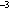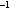U.S. Dept. of Commerce / NOAA / OAR / PMEL / Publications

##Figure 1. Winter (Jan–Mar in the north and Jul–Sep in the south) surface outcrops ofn = 25.0 and 26.5 kg mand the pycnocline base (dashed lines) from seasonal climatologies of temperature (Levitus and Boyer 1994) and salinity (Levitus et al. 1994).

Figure 2a. Maps of properties onn = 25.0 kg m, within the tropical pycnocline. Color palettes are consistent for Figs. 2–5 except for acceleration potential, which has a varying offset. The locations of the mean hydrographic profiles (black dots) are given by the average positions of the CTD stations within bins mentioned in section 2. All panels are objectively mapped from values at these locations. (a) Depth, contour intervals of 25 m. (b) Acceleration potential relative to 900 dbar, contour intervals of 0.25 J kg.

Figure 2b. (c) Salinity, contour intervals of 0.1 (PSS-78) . (d) Planetary potential vorticity, contour intervals of 200 × 10ms.

Figure 3a. Maps of properties onn = 26.5 kg m, below the tropical pycnocline. Details follow Fig. 2. (a) Depth, contour intervals of 25 m. (b) Acceleration potential relative to 900 dbar, contour intervals varying from 0.1 to 0.25 J kg.

Figure 3b. (c) Salinity, contour intervals of 0.1 (PSS-78). (d) Planetary potential vorticity, contour intervals of 25 × 10ms.

Figure 4. Mean meridional-vertical sections of salinity contoured at 0.1 intervals (PSS-78) with contours ofn at varying intervals (thick lines 25.0, 26.0, and 26.5 kg m) overlaid in white. Color palettes follow Fig. 2. Nominal vertical exaggeration is 5000:1. (a) 165°E. (b) 125°W.

Figure 5. Mean meridional-vertical sections of planetary potential vorticity contoured at logarithmic intervals (10ms) with contours ofn at varying intervals (thick lines 25.0, 26.0, and 26.5 kg m) overlaid in white. Color palettes follow Fig. 2. Nominal vertical exaggeration is 5000:1. (a) 165°E. (b) 125°W.

Figure 6. Net meridional mass transport (10kg s) excluding the mixed layer binned inn every 0.2 kg mfrom the South American coast to (a) 165°E at 8°S and (b) 135°E at 8°N. In the south, equatorward flow is concentrated near the eastern STMW density and poleward flow belown = 26.1 kg m. In the north, equatorward flow is at lighter densities and poleward flow belown = 25.9 kg m. (c) Downward accumulated integrals of (a) and (b) show the net interior transport within the pycnocline (ton roughly 26 kg m) in the northern hemisphere (6 × 10kg s) is much less than that in the southern hemisphere (14 × 10kg s).

Figure 7. Quasi-meridional interior pycnocline (mixed-layer base to pycnocline base) mass transport (10kg s) zonally accumulated from the Americas westward. Symbols are individual integration points and lines are objectively mapped assuming a Gaussian covariance with a correlation length scale of 20° longitude and an error-to-signal energy of 0.25. (a) Southern hemisphere transports at three latitudes (17°S solid line, 12°S dashed, and 7°S dot-dashed) with a pycnocline base atn = 26.2 kg m. (b) Northern hemisphere transport between the NEC and NECC with a pycnocline base atn = 25.9 kg m. (c) Northern hemisphere transport between the NECC and SEC with a pycnocline base atn = 25.9 kg m.

Table 1. Latitudinal range, maximum neutral density, and maximum pressure of the quasi-zonal tropical currents at 165°E and 125°W (see Fig. 4).

Go to Abstract

PMEL Outstanding Papers

PMEL Publications Search

PMEL Homepage